# Find duplicate data in MySQL

## Objective

There are many occasions when you need to find duplicate values available in a column of a MySql table. Often, you may want to count the number of duplicate values in a MySQL table.

In this article, we have discussed a query where you can find duplicates, triplicates, quadruplicates (or more) data from a MySQL table.

We have discussed how to find duplicate values with INNER JOIN and subquery, INNER JOIN and DISTINCT, and also how to count duplicate values with GROUP BY and HAVING.

Table in question

We have used a table called 'item' to apply the query :
Table Name: item
Structure: item_code varchar(20), value int(11), quantity int(11) where item_code is the primary key.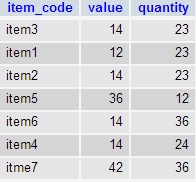Using INNER JOIN and Subquery

Now we want to get the details of those records where quantity field have duplicate/triplicates values. In the image above, values marked with red rectangle exist more than once.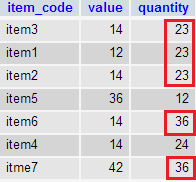Here is the query:

Code:

``````SELECT item_code, value, item.quantity
FROM item
INNER JOIN(
SELECT quantity
FROM item
GROUP BY quantity
HAVING COUNT(item_code) >1
)temp ON item.quantity= temp.quantity;
```
```

Sample Output:To get the above result we have used a query with an INNER JOIN (INNER JOIN selects all rows from both participating tables as long as there is a match between the columns.) statement. INNER JOIN uses the main table 'item' and a temporary table 'temp' whose data comes from a subquery. Here is the subquery and it's output:

Code:

``````SELECT quantity
FROM item
GROUP BY quantity
HAVING COUNT(item_code) >1
```
```

Sample Output: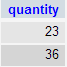Now the following main query will execute on 'item' and 'temp' tables where the common field is quantity and the result will be as follows:

Code:

``````SELECT item_code, value, item.quantity
FROM item
INNER JOIN temp ON item.quantity= temp.quantity;
```
```

Using INNER JOIN and DISTINCT

You can use the following query to get the same result. Here we apply INNER JOIN the table with itself. As the same quantity value exists in more than two records, a DISTINCT clause is used.

Here is the code and the output :

Code:

``````SELECT distinct a.item_code, a.value, a.quantity
FROM item a
INNER JOIN item b ON a.quantity = b.quantity
WHERE a.item_code <> b.item_code
```
```

Sample Output: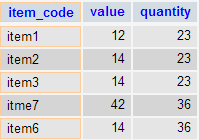Count duplicate data in MySQL

The following query count those records where quantity field holds duplicate/triplicates (or more) data.

Table data:Code:

``````SELECT item_code, COUNT( quantity ) x
FROM item
GROUP BY quantity
HAVING x >1
```
```

Sample Output: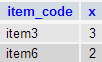Count duplicate records in MySQL

To count the total duplicate (or more) 'quantity' of 'item' table you can use the following query:

Code:

``````SELECT count(*) AS Total_duplicate_count
FROM
(SELECT item_code FROM item
GROUP BY quantity HAVING COUNT(quantity) > 1
)AS x
```
```

Sample Output: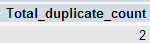﻿